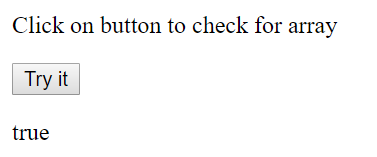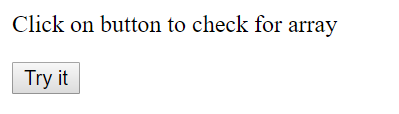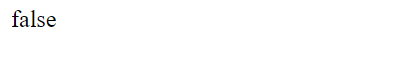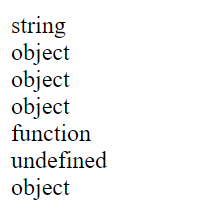# How to check object is an array in JavaScript?

Method 1: Using Array.isArray() function: The Array.isArray() function determines whether the value passed to this function is an array or not. This function returns true if the argument passed is array else it returns false.

Syntax:

`Array.isArray(obj)`

Here, obj is any valid object in JavaScript like map, list, array, string, etc.

Return Value: It returns Boolean value true if the object passed is an array or false if the object passed is not an array.

Example 1: This example uses Array.isArray() function to check the object is array or not.

 ` ` `<``html``> ` ` `  `<``head``> ` `    ``<``title``> ` `        ``check object is an array ` `    `` ` ` ` ` `  `<``body``> ` `    ``<``p``> ` `        ``Click on button to ` `        ``check for array ` `    `` ` `     `  `    ``<``button` `onclick``=``"myFunction()"``> ` `        ``Try it ` `    `` ` `     `  `    ``<``p` `id``=``"GFG"``> ` `     `  `    ``<``script``> ` `        ``function myFunction() { ` `            ``var countries = ["India", "USA", "Canada"]; ` `            ``var x = document.getElementById("GFG"); ` `            ``x.innerHTML = Array.isArray(countries); ` `        ``} ` `    `` ` ` ` ` `  `                     `

Output:Example 2: This example uses Array.isArray() function to check the object is array or not.

 ` ` ` ` ` `  ` ` `    `` ` `        ``check object is an array ` `    `` ` ` ` ` `  ` ` `    ``

` `        ``Click on button to ` `        ``check ``for` `array ` `    ``

` `     `  `    `` ` `     `  `    ``

` `     `  `    `` ` ` ` ` `  `                     `

Output:

• Before Clicking the button:• After Clicking the button:Example 3: This example uses Array.isArray() function to check the object is array or not.

 ` ` ` ` ` `  ` ` `    `` ` `        ``check object is an array ` `    `` ` ` ` ` `  ` ` `    ``

` `        ``Click on button to ` `        ``check ``for` `array ` `    ``

` `     `  `    `` ` `     `  `    ``

` `     `  `    `` ` ` ` ` `  `                     `

Output:

• Before Clicking the button:• After Clicking the button:Method 2: Using typeof operator: In JavaScript, the typeof operator returns the data type of its operand in the form of a string where an operand can be any object, function or variable. However, the problem with this is that it isn’t applicable for determining array.

Syntax:

`typeof operand or typeof(operand)`

Example:

 ` ` `<``html``> ` ` `  `<``head``> ` `    ``<``title``> ` `        ``check object is an array ` `    `` ` ` ` ` `  `<``body``> ` `    ``<``p` `id``=``"GFG"``>  ` `     `  `    ``<``script``>  ` `        ``document.getElementById("GFG").innerHTML ` `          ``= typeof "Geeks" + "<``br``>" +  ` `            ``typeof [1, 2, 3, 4] + "<``br``>" +  ` `            ``typeof {name:'Kartik', age:20} + "<``br``>" +  ` `            ``typeof new Date() + "<``br``>" +  ` `            ``typeof function () {} + "<``br``>" +  ` `            ``typeof job + "<``br``>" +  ` `            ``typeof null;  ` `    ``  ` `  ` ` `  `                     `

Output:My Personal Notes arrow_drop_upCheck out this Author's contributed articles.

If you like GeeksforGeeks and would like to contribute, you can also write an article using contribute.geeksforgeeks.org or mail your article to contribute@geeksforgeeks.org. See your article appearing on the GeeksforGeeks main page and help other Geeks.

Please Improve this article if you find anything incorrect by clicking on the "Improve Article" button below.